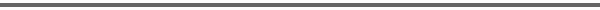Journal Home Page Complete Contentsof this Volume Previous Article Next Article Journal of Lie Theory 14 (2004), No. 1, 111--140 Copyright Heldermann Verlag 2004Algorithmic Construction of Hyperfunction Solutions to Invariant Differential Equations on the Space of Real Symmetric Matrices Masakazu Muro Gifu University, Yanagito 1-1, Gifu, 501-1193, Japan, muro@cc.gifu-u.ac.jpThis is the second paper on invariant hyperfunction solutions of invariant linear differential equations on the vector space of n x n real symmetric matrices. In a preceding paper [J. Funct. Anal. 193 (2002) 346--384], we proved that every invariant hyperfunction solution is expressed as a linear combination of Laurent expansion coefficients of the complex power of the determinant function with respect to the parameter. Fundamental properties of the complex power have been investigated by the author in the paper "Singular invariant hyperfunctions on the space of real symmetric matrices" [Tohoku Math. J. 51 (1999) 329--364]. In this paper, we give algorithms to determine the space of invariant hyperfunction solutions and apply the algorithms to some examples. These algorithms enable us to compute in a fully constructive way all the invariant hyperfunction solutions for all the invariant differential operators in terms of Laurent expansion coefficients of the complex power of the determinant function. Keywords: invariant hyperfunctions, symmetric matrix spaces, linear differential equations. MSC 2000: 22E45, 58J15, 35A27. [ Fulltext-pdf  (314  KB)] for subscribers only.